# Absolute Permeability

Absolute permeability is an ability to flow fluid through a permeable rock when only one type of fluid is in the rock pore spaces.  The absolute permeability is used to determine relative permeability of fluids flowing simultaneously in a reservoir.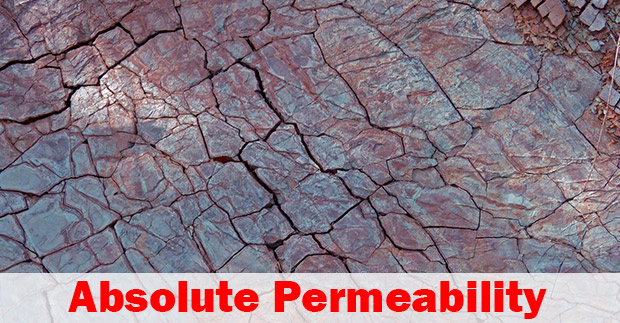Darcy’s equation is widely used in an oil field to measure flow in porous media and the Darcy’s linear flow equation is shown below;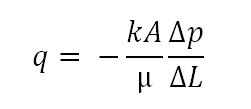Equation 1 – Darcy’s Equation for Linear Flow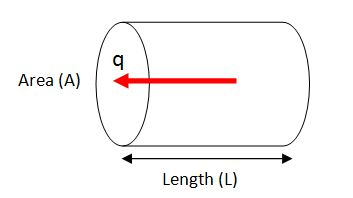Figure 1 – Liner Flow Diagram

Where;

q = flow rate, cc/sec

k = permeability, Darcy (D)

A = cross sectional area to flow, cm2

μ = fluid viscosity, cp

Δp = pressure, atm

ΔL = length of fluid path, cm

For oilfield unit, the equation above is expressed below;Where;

q = flow rate, stb/d

k = permeability, milli Darcy (mD)

A = cross sectional area to flow, ft2

μ = fluid viscosity, cp

Δp = pressure, psi

ΔL = length of fluid path, ft

Note: These equations (Equation 1 and Equation 2) represent a linear flow and a minus sign is in the equation because Δp is a minus figure so these two minus signs can cancel each other.

From the Equation 2, permeability can be expressed like this.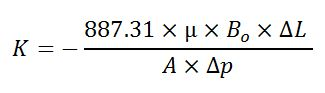Where;

q = flow rate, stb/d

k = permeability, milli Darcy (mD)

A = cross sectional area to flow, ft2

μ = fluid viscosity, cp

Δp = pressure, psi

ΔL = length of fluid path, ft

Note: For a radial flow, we will go into details in later articles.

In 3-dimensional flow, permeability of each axis of low represents by the following signs;

kx – the permeability in the x-direction (horizontal permeability)

ky – the permeability in the y-direction (horizontal permeability)

kz – the permeability in the z-direction (vertical permeability)

kx and ky are parallel to a bedding plane of formation, but kz is perpendicular to a bedding plan.

Generally, horizontal permeability and vertical permeability has a significant level of difference. The variation in permeability in different planes is called Permeability Anisotropy. In reservoir modelling, it is quite common to have kx close to ky but, kz should be significantly different.

Reservoirs with layered formation have several permeability values, so these permeability values must be average to represent the whole sand package. There are two methods used to average permeability.

Thickness Weight Average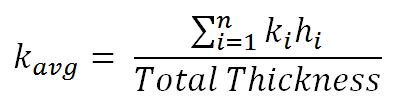Where;

kavg = average permeability

k = permeability of layer

h = thickness of layer

Geometric MeanWhere;

kavg = average permeability

How to Measure the Absolute Permeability

The absolute permeability is determined by flowing a single fluid of known viscosity through a core sample at a planned rate and different pressure. Then use Equation 1 to calculate the absolute permeability. This value will represent the answer only in a small scale because a core sample is so small compared to a reservoir’s size.

References

Abhijit Y. Dandekar, 2013. Petroleum Reservoir Rock and Fluid Properties, Second Edition. 2 Edition. CRC Press.

L.P. Dake, 1983. Fundamentals of Reservoir Engineering, Volume 8 (Developments in Petroleum Science). New impression Edition. Elsevier Science.

Tarek Ahmed PhD PE, 2011. Advanced Reservoir Management and Engineering, Second Edition. 2 Edition. Gulf Professional Publishing.

Share the joy
Tagged , . Bookmark the permalink.